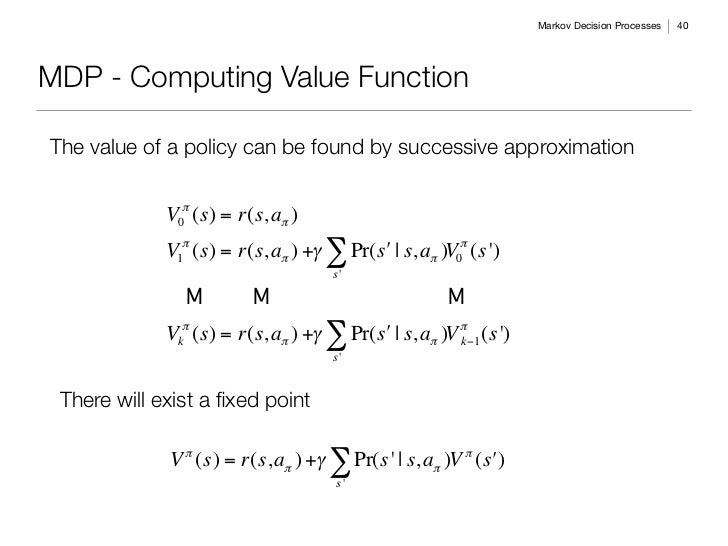# How do I choose a discount factor in Markov Decision Problems?

I'm referring to the gamma in the Value function:This is the typical value function of Reinforcement Learning. The discount factor evaluates the importance of the accumulated future events in your current value. The smaller the number, the less important are the future events in the current action.

Usually this number is selected heuristically. I usually select 0.9. If I don't want any discount then I would select 1.

• lmao at using "heuristically" to say "I just go with my gut" Nov 20 '20 at 16:41

$$\gamma$$ is a hyperparameter of the reinforcement learning algorithm, so you can apply technics for hyperparameter optimization like grid search or bayesian optimization.

There is no general recommendation possible because it depends on the problem you want to solve. But you probably will need a value near 1 if your rewards are quite sparse

Selecting the discount factor $$\gamma$$ depends on the problem. As explained by Sutton & Barto the value is always between 0 and 1: $$0<=\gamma<=1.0$$. If $$\gamma=0$$ the policy will be greedy, i.e. it will choose the best action only for the current state. And if $$\gamma>0$$ then (possible) future rewards will be taken into account. When $$\gamma<1$$ then the infinite sum is finite as long as the reward sequence is bounded.

As also commented in this related answers, with a higher $$\gamma$$ the policy is optimized for gains further in time, but will take more time to converge.

• So how do I know whether to use a .25 discount factor or a .75 one? When do I want to use a greedy gamma? Is there a formula to get precise value or do I just "use whatever feels right"? Feb 9 '16 at 4:51